# Slope

/Slope
Slope 2017-11-13T22:01:52+00:00

Slope can be a rate of change, a ratio, and is known as rise over run. It is often denoted by the letter ‘m’ in equations. It can be a decimal number but is often written as a fraction and seldom as a mixed number. The following video and explanation will help you get an understanding of slope and its many uses.

Slope is defined as rise over run. When you think of slope, think of steepness. Ski slopes come to mind as well as steep mountain roads, the pitch of a roof, or a gradual elevation change. Consider the following photos that depict varying slopes.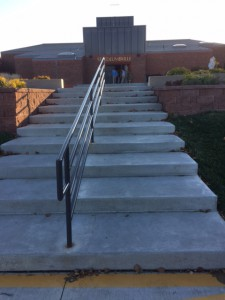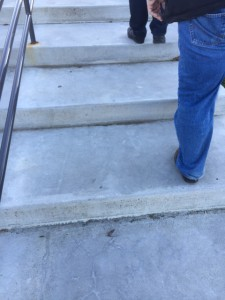These steps are difficult to climb if you only want to use each step once. They have a shallow slope. For each step that rises 6.5”, it has a depth (or run) of 28”. There are ten steps. To find the slope, simply divide the rise by the run 65 inch total rise divided by 280 inches total run. Slope is usually written as a fraction in reduced form. The slope of these stairs is 13/56.

A steeper set of stairs that is difficult to climb is pictured in this machine shop. The owner needs to have his hands free to carry things to the loft. However, he didn’t want to lose a lot of floor space to a standard stair case.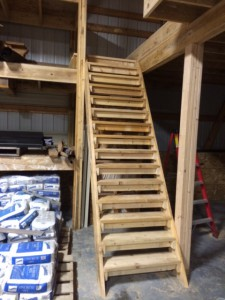The depth of tread (run) in this example is 9 ¼”  . Measure the length of your foot to see why these steps are difficult to climb. If the total rise is 10 feet and the run is 7.5 feet, what is the slope of these steps? Is it steeper or shallower than the first set of stairs?

This ladder touches the house 160 inches above the ground. Its base is 54 inches away from the house. What is its slope?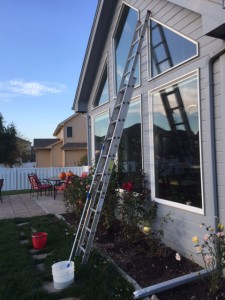Now that we have established what slope is, let’s look at two edge cases. What would the slope of a flat surface be? A football field, for example stays the same height, but has a lot of depth. It is not rising (0 rise) and has a run of more than 100 yards. What is its slope?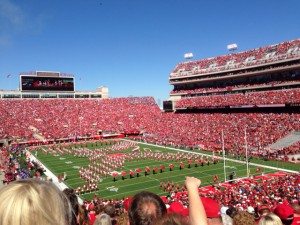Finally, look at the climbing wall. It has a lot of rise, but no run. What is its slope?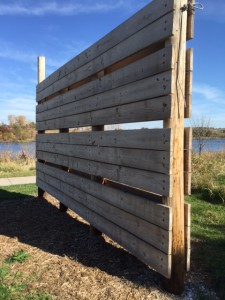Up next: Graphing Slope